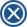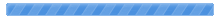Welcome, Guest. Please Login or Register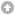CAPTAIN SIM FORUM › 757 Captain › 757 version 1.x-2.x (FS9 ONLY) › CS757 true CG weight and balance.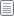Pages: 1 Send TopicPrintCS757 true CG weight and balance. (Read 3852 times)
 SEAN ZARELLA Full Member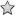OfflinePosts: 36Joined: Sep 18th, 2013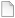CS757 true CG weight and balance. Jun 16th, 2014 at 1:20pm Print Post Good morning pilots, I have been flying the cs757 for about 3 years now, i have my own Simpit i created.I have taught myself CG load and balance, and have edited the CS757 aircraft.cfg to reflect the load station data of the real 757-200.Know that the empty cg of a 757-200 is 23%I will start with the basics on the fundamentals before i go into the actual math involved in determining your planes CG% or %mach can also be called. All Center of gravity is based upon the starting point which we call DATUM. DATUM is refered as the starting point of the line which the manufacturer determined for each plane they make, and for the B752 that line starts 159 inches infront of the nose. You draw that line linear from that point to the start of a small window of balance which we call MAC. which is mostly located at the wings ( center Of plane) So you start with your Datum and a line is drawn from there to the start of the MAC and measure that distance in inches. , the start of the MAC line we call LEMAC or leading edge MAC. and for the B752 LEMAC is @ 991.9 inches from the datum. The mac line starts from there and is 199.7 inches long,. we refer MAC as 199.7 inches. So drawing that line from Datum to the LEMAC point and if you add up the point of LEMAC 991.9 + your mac 199.7 = 1191.6 0% mac is 991.9 inches. where it starts, 100% mac is 1191.6 inches. Here is a image of what we are talking about, I illustrated where the datum line starts and where lemac is and 0 - 100 % MAC.Between 0%MAC and 100% MAC is the window of CG we need the plane to be balanced. But that isnt the balance point. Per aircraft each is different based on Weight and placement of Objects forward and aft of the LEMAC line. For instance, For the B752 The CG window envelope is 7% - 39% CG and that depends on your Gross Weight. This can be found in the 757 - 200 weight and balance handbook. Finaly, where the Cg is located within that window is called BALANCE ARM or the distance from CG% to datum. measured in inches. ARM is measured by balance point when the airplane is at DRY OPERATIING WEIGHT or EMPTY WEIGHT, and changes from aircraft to aircraft because of seating configurations forward and Aft Lemac. I found out that per the 757-200 that my Virtual Airline Flies is called.  75X PER  seating specification that can hold 184 PAX. The Balance Arm or distance from datum to the EMPTY WEIGHT CG is 1037.8 inches. This is How you determine your planes EMPTY CG% ````````````distance aft of LEMAC `` cg%mac = ___________________ ```````````````````` MAC Distance aft OF LEMAC is easy to figure out now, if our LEMAC is 991.9 inches and the balance arm is 1038.7 inches. we take 1038.7 -991.9 = 46.8 inches. ````````46.8 CG%=____________ = 23.43% ```````1.997 if you just had your CG% and needed your ARM ( to compute DOI ) ~~~~~~~~~~~~~~~~~~%MAC Balance Arm = LEMAC + ( ----------------� MAC ) ~~~~~~~~~~~~~~~~~~ 100 SO ``````````````` 23.43% ARM = 991.7 + ( ----------------- x MAC ) = 1038.68971 or 1038.7 `````````````````100 Next is Computing the planes Dry Operating Index empty weight. Formula for this is for the B752 DOI = Weight x (Arm - 1037.8) + 50 ````````````````75000 Since balance arm is 1037.8 that zeros out the equation and you add 50. so our DOI is 50. refer to the actual LOAD SHEETand finally go to your aircraft.cfg and go to the following section, copy and paste the new data. This will give you a fully loaded 184 pax 757 with 9200 cargo . As well as an Empty CG of 23% �to match the 757x [WEIGHT_AND_BALANCE] max_gross_weight = 256000 // (pounds) empty_weight = 127520 // (pounds) reference_datum_position = 2.3, 0, 0 // (feet) distance from FlightSim Reference position: (1/4 chord, centerline, waterline) empty_weight_CG_position = 2.5, 0, 0 // (feet) longitudinal, lateral, vertical distance from specified datum max_number_of_stations =12 station_load.0 ="460, 70, 0, 0, Flight Crew" station_load.1 ="920, 0, 0, 0, Cabin Crew" ;Moments of Inertia empty_weight_pitch_MOI = 7040000 empty_weight_roll_MOI = 1960000 empty_weight_yaw_MOI = 7500000 empty_weight_coupled_MOI = 1000 station_load.2=4550, 25, 1, 0, F/C Row 1-7 station_load.3=11900, 14, 1, 0, E/C ECON Row 20-30 station_load.4=9450, -13, 0, 0, ECON Row 31-39 station_load.5=6300, -33, 0, 0, ECON Row 40-45 station_load.6=888, 25, -1, -2, FWD Cargo Bay 1 station_load.7=0, 12, -1, -2, FWD Cargo Bay 2 station_load.8=8318, -12, -1, -2, AFT Cargo Bay 3 station_load.9=0, -25, -1, -2, AFT Cargo Bay 4 station_load.10=1975, 0, 0, 0, OPERATIONAL ITEMS station_load.11=0, 0, 0, 0, ADDITIONAL WEIGHT Ok so testing this Mod, Your ZFW CG % will be 30% Your TOW CG % is now 29% and using the fmc will give trim value around 5.0 up. Thank you all for watching any questions please dont hesitate to ask.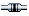IP LoggedPages: 1 Send TopicPrint

 « Board Index ‹ Board  ^Top